Dynamic Algebra in EpsilonWriter

This document contains the demonstrations made during the communication at CADGME 2014.

Additional lines are in blue and red. They indicate settings and actions to perform.

The demos were made with EpsilonWriter 2.401

Later versions may have a different behavior due to evolution of the software.

If you have this document as an HTML page, as it contains EpsilonWriter data, you can copy the document (Ctrl+A then Ctrl+C) and paste in EpsilonWriter to get the document with editable formulas. You can then apply the suggested actions.

Otherwise, you can get the html page at http://www.epsilonwriter.com/TMF/

## 1. Equivalent drag and drop

Pedagogical dynamic algebra: only basic steps

With the tab "Calculation", choose "Dynamic Algebra: Pedagogical" and "Explain calculations on the line".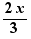Move 2 in front of the fraction: this is a possible basic movement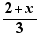Move 2 in front of the fraction: this is not a possible basic movement

2x=3

Move 2 on 3 : this is a possible basic movement

=============================================

Calculation dynamic algebra: more powerful

With the tab "Calculation", choose "Dynamic Algebra: Strong".

Solve 2(x+2)=3

Move 2 on x+2 then 4 on 3 then 2 on -1

Factorize (x-3)(3x+2)+x(9x+6)

Move 9 in front of x and choose "Factoring HCF". Move (3x+2) in front of (x-3) .  Move 3x on x

Simplify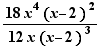Move 18 on 12 ,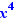on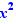and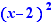on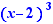Solve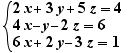Write a 3 on the line after. Move this 3 on the = sign of the second equation, choose "Multiplication of both sides". Select the first equation and move it on the = sign of the second equation, choose "Addition side with side". Solve the second equation for z by moving 14x then the - sign. Select the second equation and move it on the = sign of the first equation, choose "Substitution". Do a Ctrl+Click on the = sign of the first equation.

## 2. External drag and drop

Substitution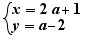Select the above simultaneous equalities and move them on the fraction bar below. Ctrl+Click on the fraction bar.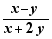=============================================

## 3. Completing the square and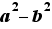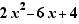Select the expression and choose "Completing the square". Select the first 2 and move it a little to the left to factor out 2 . Select the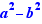form and choose "Factorization with". Ctrl+Click on the adequate - and + signs to add the numerical fractions.

## 4. Solving schemas

Solve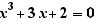Select the equation and choose "Solve equation". You get the Cardan's formulas solving schema. Move the p= and q= on the = sign of Δ=  and choose "Substitution". Use Ctrl+Click to calculate Δ.  As Δ>0 , click on "ok" for the case Δ>0 and on the delete buttons for the two other cases. Select Δ=8 and move it on the = sign of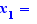, choose "Substitution". Ctrl+Click on the inner radicals to transform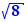into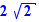. Select q=2 and  move it on the = sign of. Ctrl+Click on the two fraction bar.

## 5. Suggestions

Solve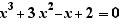Select the equation and choose "Solve equation". Select x=y-1 and move on the = sign of the equation below,  choose "Substitution". Ctrl+Click on the = sign of the obtained equation to expand and simplify. You are now in the situation 4.

## 6. Tables of signs and solutions of an inequality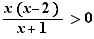Select the inequality and choose "Table of signs filled and solution".

Select again the inequality and choose "Table of signs to fill".

## 7. Definition conditions of a function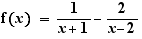Ctrl+Click on the = sign to get the definition condition then Ctrl+Click on and to get a definition set.

## 8. Limits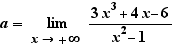Ctrl+Click on lim  to get the application of a theorem of "highest degrees". Move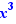onto get a simplification.  Ctrl+Click on lim  to get the result.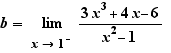Ctrl+Click on lim  to apply the substitution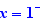Select the numerator and choose "Numerical calculation".  Select the denominator and choose "Numerical calculation". You get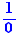which is  an indefinite expression. Delete this step by Ctrl+Z then select the denominator and choose "Calculation of a limit". You get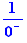.  Ctrl+Click on the fraction bar, you get the result.

## 9. Derivatives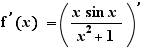Ctrl+Click on the prime on the right of the = sign. Repeat this action until there is no prime.

## 10. Variation tables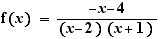Duplicate the formula (use the "Duplicate" button or copy and paste). Put a prime on f. Select the fraction and type prime.

Ctrl+Click on the prime on the right of the = sign. Repeat this action on the prime obtained.  Select the numerator and expand and simplify. Select the exponent 2 of the denominator and move it through one parenthesis on the right. Select the numerator and apply "Completing the square". Select the numerator and apply "Factorization with". You get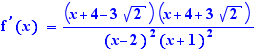Ctrl+Click on the  = sign, you get the "Variation table". Remains to fill the limits and two values of the function.

## 11. Several presentations of the explanations

-2x>3

Select -2 and move it over 3.

With the tab "Calculation", choose "Explain calculations in a table".

Go back to the given inequality, select -2 and move it over 3.

With the tab "Calculation", choose "Explain calculations in a table".

Select -2 and move it over 3. There is now a mathematical explanation and a gesture description.